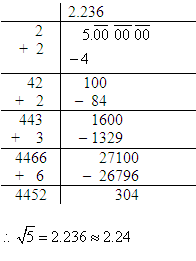# Square Root of 5

The square root of 5 belongs to a long list of irrational algebraic number. It has been categorized in such list because the square root of 5 cannot be expressed as a fraction and has an infinite number of decimals.

The square root of 5 is usually written as √5 in mathematics and is said to be the positive algebraic number which is multiplied by itself will give the prime number 5. It is more accurately called the principal square root of 5, and this is basically to distinguish itself from the negative number with similar property.

Like √2 and √3, the square root of 5 also appears extensively in the formulae for exact trigonometric constants. It also appears in several other instances. Geometrically, √5 is said to be the diagonal of a rectangle having side length of 1 and 2. This is yet again proved by the Pythagorean Theorem.

## What is the Square Root of 5?

The numerical value of the square root of 5, which has been shortened to 50 decimal places is as follows: 2.23606797749978969640917366873127623544061835961152… This is the simplified value of square root of 5. Interestingly, data from December 2013 reveals that the numerical value of √5 in decimal has been computed to be at least ten billion digits.

## How to Find the Square Root of 5?

If you have been wondering how to find the square root of 5, the easiest way you can do so is by the division method. In this method, you have to first make an estimate, meaning you have chosen a close number by finding at-least two square roots which are perfect. It has been those square root that your number is between. Then, you can divide the number by one of those square roots. Once you start and continue with the division process, you will need to take the average of the result of step 2 and the root. And finally, you can use the result that you get in step 3. Repeat the 2nd and 3rd step until you get a number that is satisfactory or accurate enough for you. The example is given below:Additionally, you can also try our square root calculator, which is an online tool that shows the square root for any given input. BYJU’S square root calculator makes calculations easy and interesting. You just need to put the input as 5, and then the tool quickly shows the result for the given number.

Apart from this, there are a lot of ways you can find the square of any number. To find out more, you can check out BYJU’S YouTube channel to learn the methods.Test your Knowledge on Square Root of 5